## 1. 摘要

DeeplabV1&V2 - 带孔卷积(atrous convolution), 能够明确地调整filters的接受野(field-of-view)，并决定DNN计算得到特征的分辨率；
DeeplabV3 - 多尺度(multiple scales)分割物体，设计了串行和并行的带孔卷积模块，采用多种不同的atrous rates来获取多尺度的内容信息；
DeeplabV3 - 提出 Atrous Spatial Pyramid Pooling(ASPP)模块, 挖掘不同尺度的卷积特征，以及编码了全局内容信息的图像层特征，提升分割效果；
DeeplabV3 - 无需DenseCRF后处理.

## 2. Introduction

Atrous convolution:
Atrous convolution, 即dilated convolution, 它通过移除最后几层的下采样操作以及对应filter 核的上采样操作，来提取更紧凑的特征，相当于在不同的 filter权重间插入 holes;

Atrous convolution, 决定了DCNNs计算的特征的分辨率，而不增加新的额外学习参数.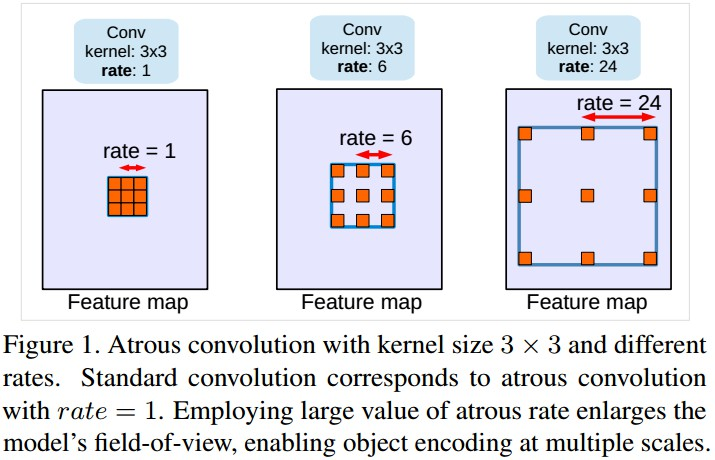Multiple scales 常见的多尺度处理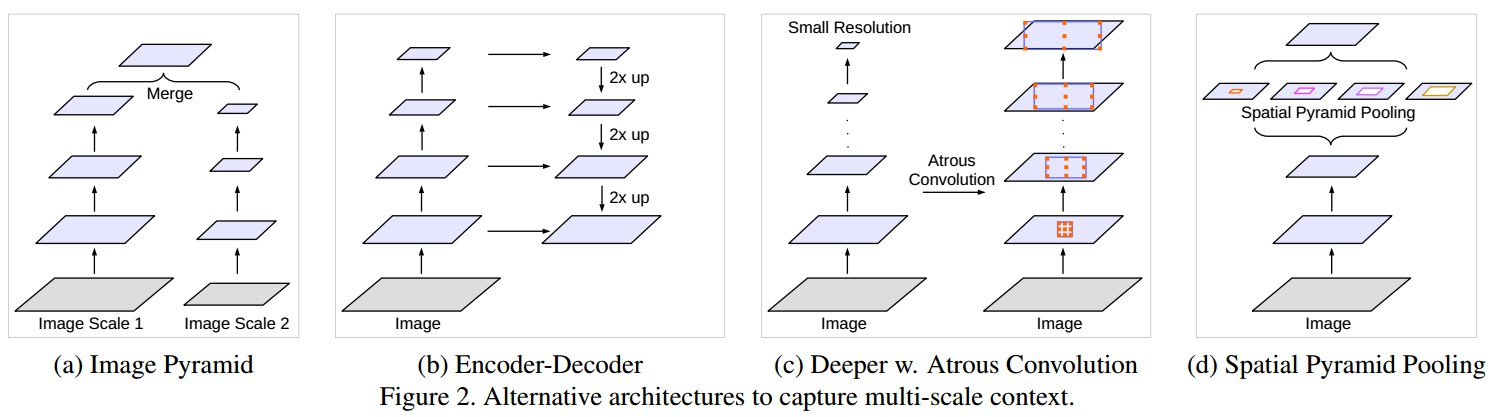DeeplabV3

## 3 DeepLabV3 Methods

### 3.1 Atrous Convolution for Dense Feature Extraction

${ y[i]=\sum_{k}x[i + r*k]w[k] }$

### 3.2 Going Deeper with Atrous Convolution

• 复制ResNet的最后一个block，如Figure3的 block4，并将复制后的blocks以串行方式级联；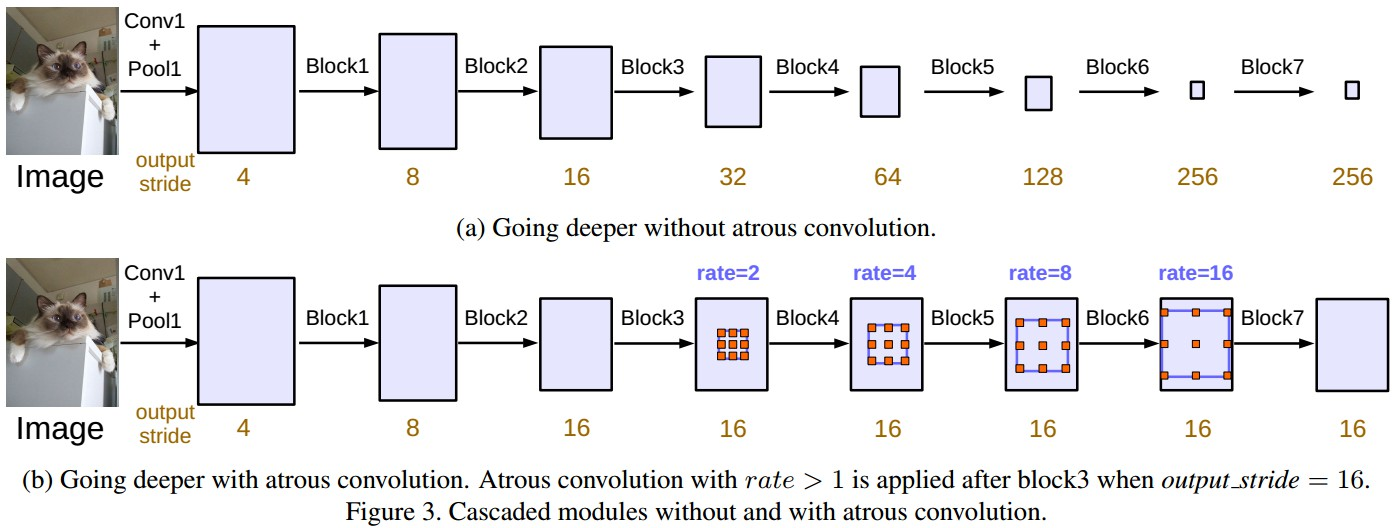• 各block中有三个convolutions， 除了最后一个block， 其它block的最后一个convolution的步长都为2， 类似于原来的ResNet.

#### 3.2.1 Multigrid

convolutional layer 最终的 atrous rate 等于 unit rate 与对应的 rate 的乘积. 例如，当 output_stride=16， Multi_Grid=(1,2,4)时, block4 中三个 three convolutions 的 rate 分别为：rates=2*(1,2,4) = (2,4,8).

### 3.3 Atrous Spatial Pyramid Pooling

ASPP, 采用四个并行的不同 atrous rates 的 atrous convolutions对 feature map 进行处理，灵感来自 spatial pyramid pooling.
ASPP, deeplabv3 中，将 batch normalization 加入到 ASPP模块.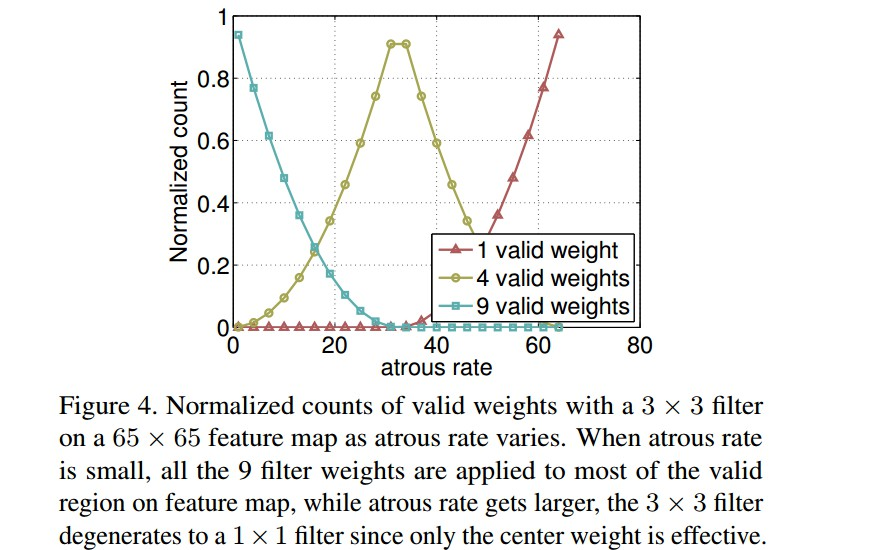(a) 当 output_stride=16时，包括一个 1×1 convolution 和三个3×3 convolutions，其中3×3 convolutions的 rates=(6,12,18)，(所有的filter个数为256，并加入batch normalization). 需要注意的是，当output_stride=8时，rates将加倍.
(b) 图像级特征, 如 Figure5.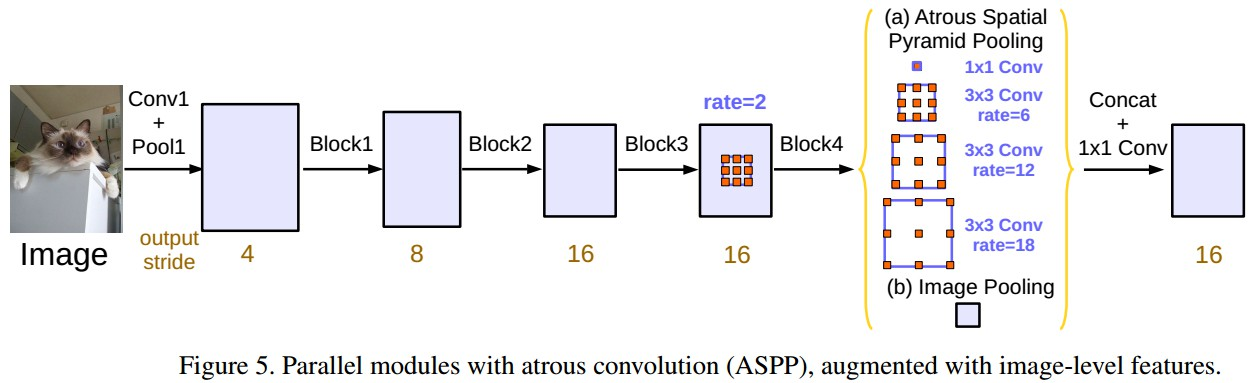## results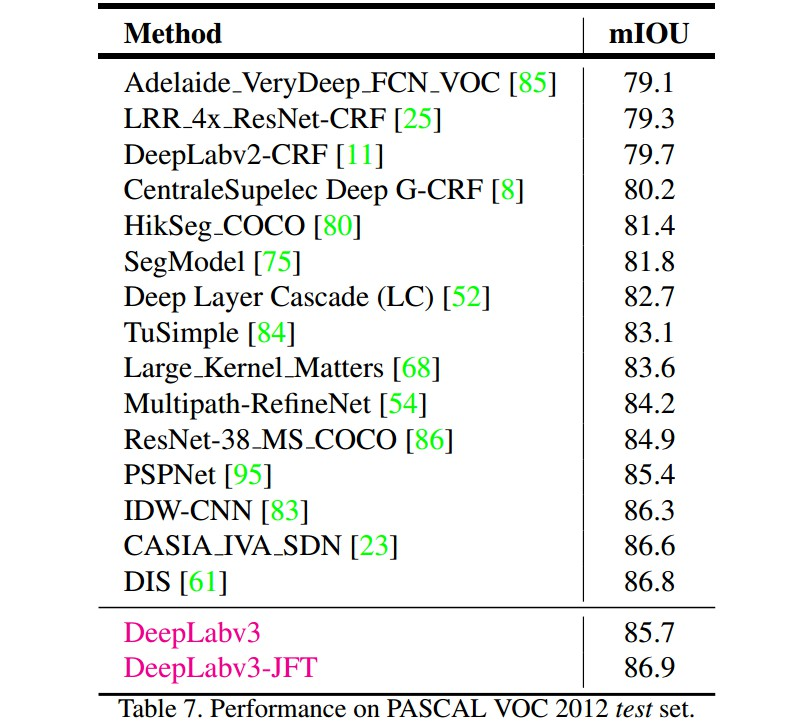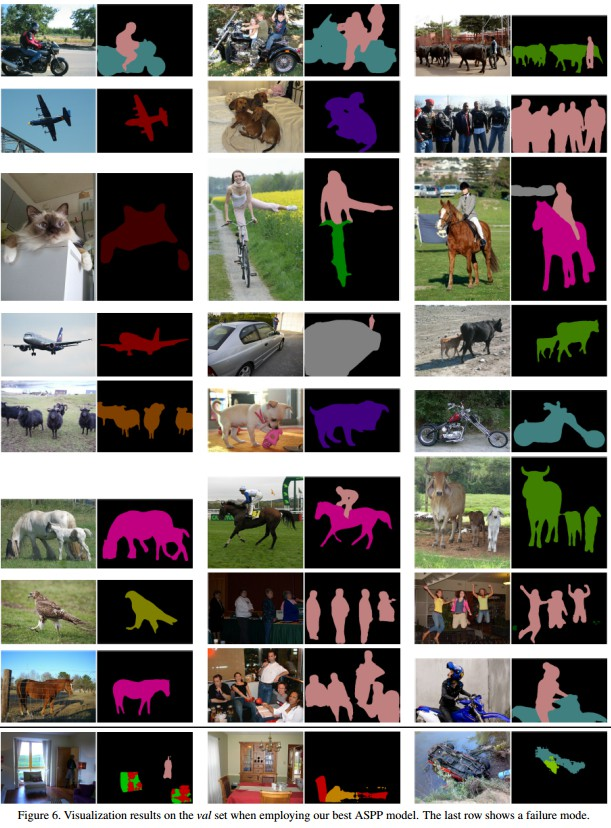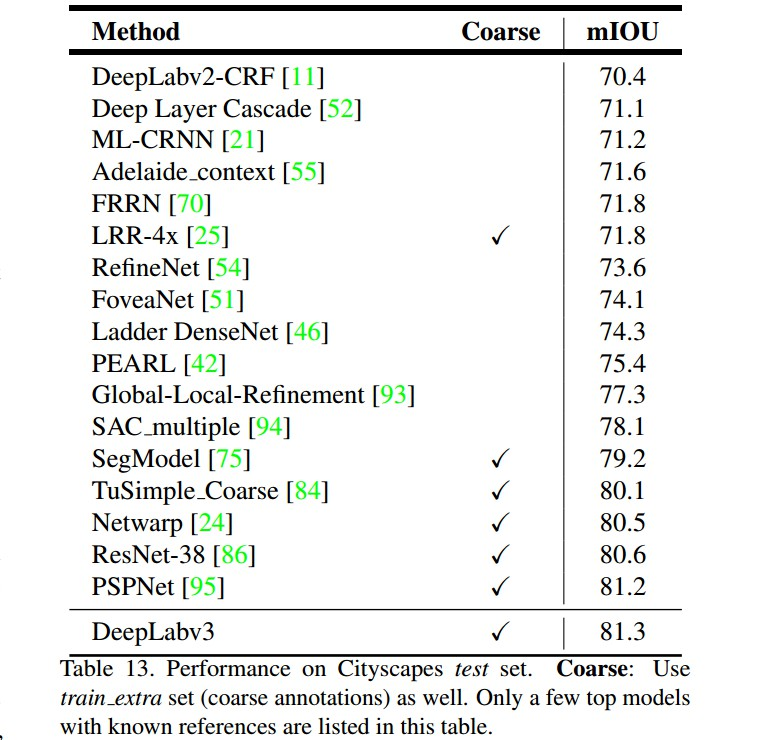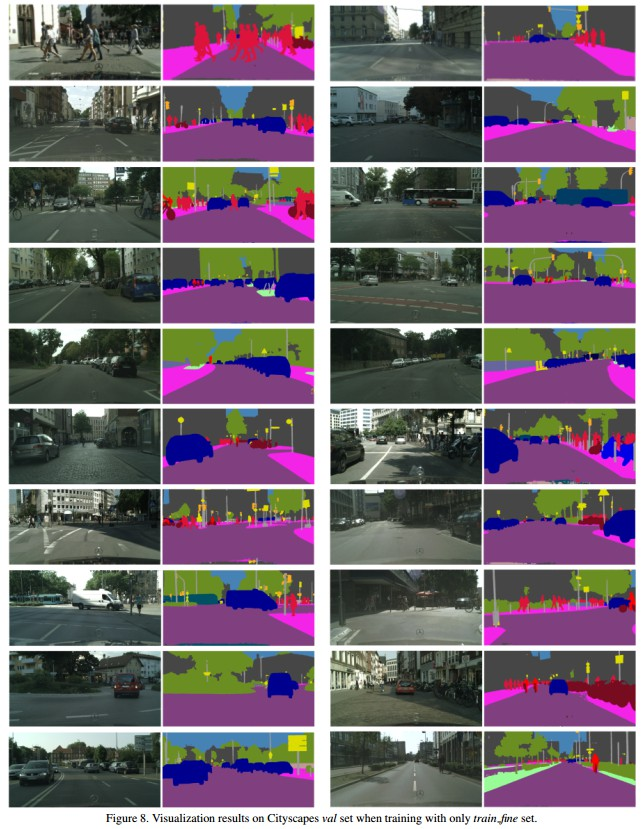Last modification：October 10th, 2018 at 01:45 am